# How to recover the Borrow Bit

In a similar way as the carry bit for addition it is possible to recover the borrow bit for substraction, just based on the highest bits of three numbers that we deal with during the operation.

With this program, a subtraction operation of an 8-bit CPU can be simulated exhaustively

 #!/usr/bin/perl

 my $x,$x, $bi; my %tab = (); for ($bi = 0; $bi <= 1;$bi++) {     for ($x = 0;$x < 256; $x++) { for ($y = 0; $y < 256;$y++) {             my $zz =$x - $y -$bi;             my $b =$zz < 0 ? 1 : 0;             my $c = 1 -$b;             my $z = ($zz + 256) & 0xff;             my $xs =$x >> 7;             my $ys =$y >> 7;             my $zs =$z >> 7;             my $key = "$xs:$ys:$zs";             $tab{$key} //= $b; my$bb = $tab{$key};             if ($bb !=$b) {                 print "b=$b bb=$bb c=$c xs=$xs ys=$ys zs=$zs x=$x y=$y z=$z zz=$zz bi=$bi\n"; } } } }  for my$key (sort keys %tab) {     $key =~ m/(\d+):(\d+):(\d+)/;$xs=$1;$ys=$2;$zs=$3;$b =$tab{$key};     $c = 1 -$b;     $bb =$xs & $ys &$zs | !$xs & ($ys | $zs); print "b=$b bb=$bb c=$c xs=$xs ys=$ys zs=\$zs\n"; } 

This gives an idea, what is happening. But in real life, probably a 64bit-CPU is used, but the concepts would work with longer or shorter CPU words the same way.

So we subtract two unsigned 64-bit integers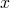and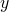and an incoming borrow bit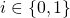to a result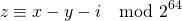with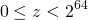using the typical „long long“ of C. We assume that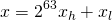where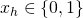and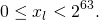In the same way we assumeand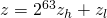with the same kind of conditions for,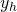,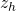or,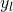,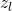, respectively.

Now we have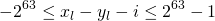and we can see thatfor some.
And we have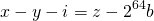where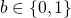is the borrow bit.
When combining we get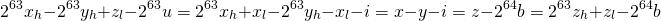When looking just at the highest visible bit and the borrow bit, this boils down to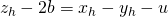This leaves us with eight cases to observe for the combination of,and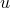:

x_hy_huz_hb
00000
00111
01011
01101
10010
10100
11000
11111

Or we can check all eight cases and find that we always haveSo the result does not depend onanymore, allowing to calculate the borrow bit by temporarily casting,and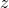to (signed long long) and using their sign.
We can express this as „use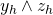if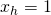and use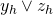if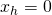„.

The incoming borrow bitdoes not change this, it just allows for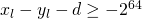, which is sufficient for making the previous calculations work.

The basic operations add, adc, sub, sbb, mul, xdiv (div is not available) have been implemented in this library for C. Feel free to use it according to the license (GPL). Addition and subtraction could be implemented in a similar way in Java, with the weirdness of declaring signed longs and using them as unsigned. For multiplication and division, native code would be needed, because Java lacks 128bit-integers. So the C-implementation is cleaner.